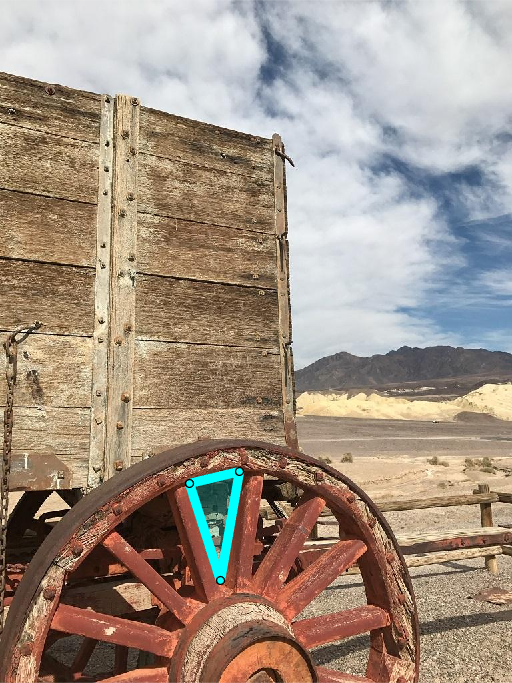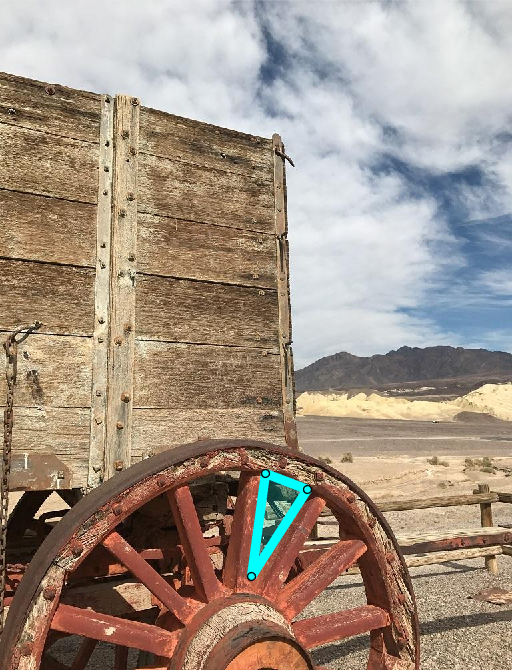# draw

Package: images.roi

Begin drawing ROI interactively

## Syntax

``draw(ROI)``
``draw(ROI,s)``
``draw(ROI,pos)``

## Description

example

``` `draw(ROI)` enters interactive mode to draw the shape for object `ROI` in the current axes (`gca`).```
``` `draw(ROI,s)` enters interactive mode to draw the shape for an `Cuboid` object, snapping the ROI to the nearest location to the mouse from the `Scatter` object `s`.```
``` `draw(ROI,pos)` enters interactive mode to draw the shape for the `Cuboid` object, snapping to the nearest location to the mouse from the position specified by `pos`. Specify `pos` as an N-by-3 numeric array where each row represents the (x,y,z) location of a potential placement position. ```

## Examples

### Draw ROI Interactively

Read an image into the workspace and display it.

```I = imread('wagon.jpg'); figure imshow(I);```Draw a triangular ROI on the image interactively. To improve the visibility of the ROI edge, specify a thick line width and bright cyan color of the ROI edge.

`p = drawpolygon('LineWidth',7,'Color','cyan');`Get the coordinates of the vertices.

`p.Position`
```ans = 284.7500 725.5000 331.2500 871.0000 359.7500 707.5000```
The spokes of the wheels define many other triangles. Suppose you want to get the vertices of a second triangle. You can use the `draw` function to start over and begin drawing a new polygonal ROI interactively. The line width and color parameters of the ROI are preserved.
`draw(p)``p.Position`
```ans = 398.7500 710.5000 377.7500 865.0000 461.7500 734.5000```

## Input Arguments

collapse all

Region of interest, specified as an ROI object of one of the following types:

Scatter plot, specified as a `matlab.graphics.chart.primitive.Scatter` object.

Position of ROI, specified as an N-by-3 numeric array where each row represents the (x,y,z) location of a potential placement position.